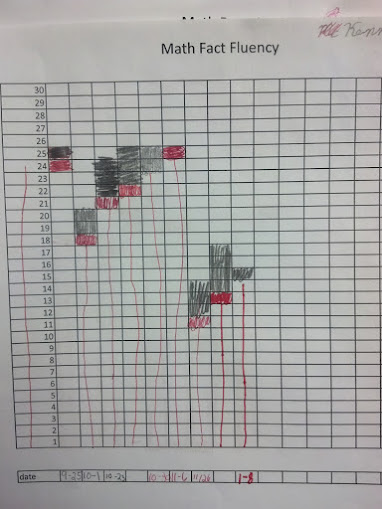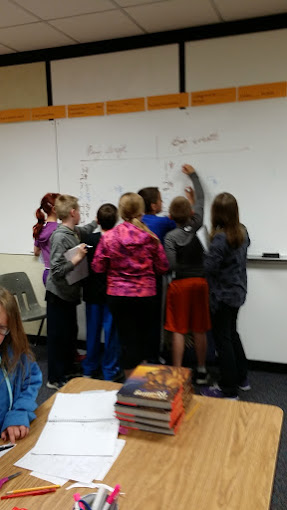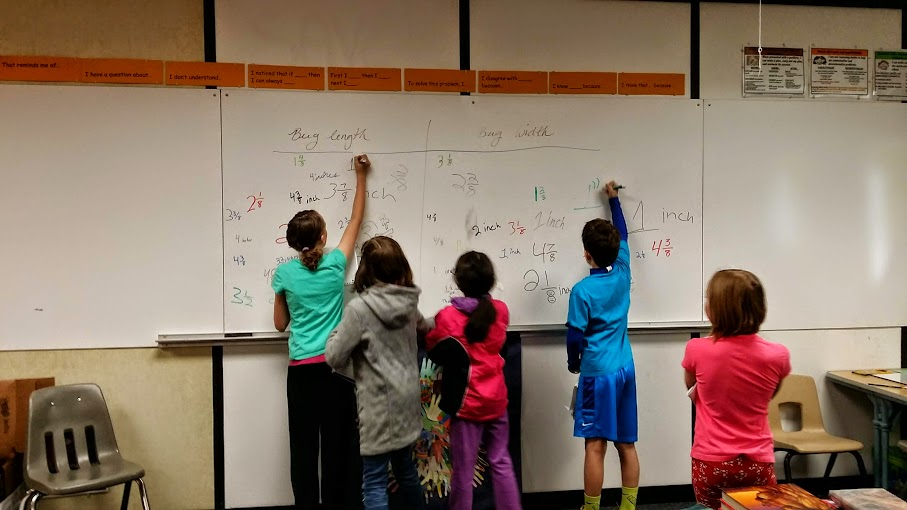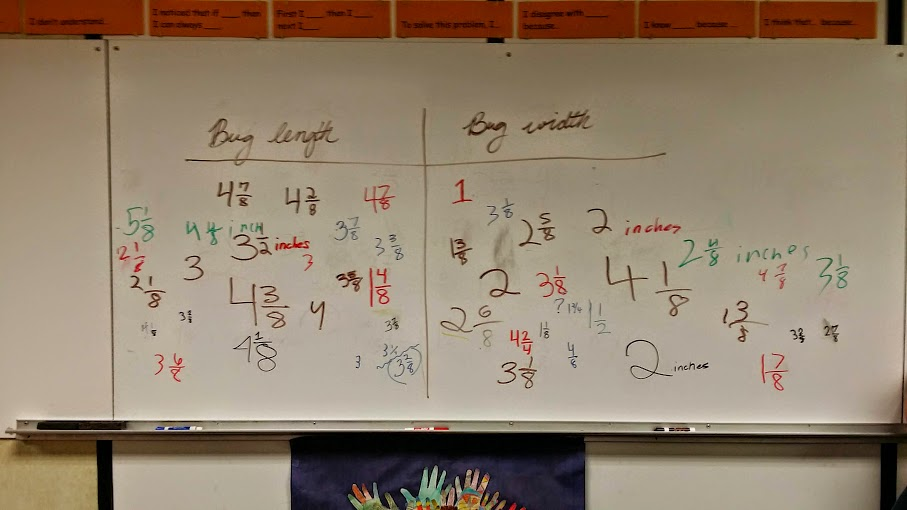# Pencils, Bugs, and Line Plots! - Day 2

5 teachers like this lesson
Print Lesson

## Objective

SWBAT create line plots to show measurements involving fractions as well as use the four operations to solve word problems involving measurement; including simple fractions and decimals.

#### Big Idea

In this multi-day lesson, students work with partners to construct line plots.

## Warm Up

7 minutes

Students will start today's lesson with a fluency assessment.  This assessment is from Monitoring Basic Skills Progress Second Edition: Basic Math Computation by Lynn S. Fuchs, Carol L. Hamlett, and Douglas Fuchs.

This is an assessment I have my students do each week and then graph their results.  It allows them to reflect on their learning of basic math facts, as well as using all four operations with whole numbers, and adding and subtracting unit fractions.  (It also happens to be the quietest time in my math classroom all week!!)

This is what my classroom looks like as students work on this assessment.

This is sample of a student's graph. You can also click here to hear my thoughts about this graph below.Click hereto see an example of a typical fourth grade fluency decrease since students are completing the fourth grade fluency set that incorporates division, multiplication, addition, subtraction, and adding and subtracting fractions with like denominators. (At this point, students have not had many fraction lessons, thus very very students are able to complete the fraction problems)

I do not start my students with the fourth grade skills, but at this point in the year, all students are using the fourth grade set.. I chose to start them with the end of the third grade skills which covers addition, subtraction and multiplication and division of basic facts. I strongly believe in a balanced math approach, which is one reason why I also believe in common core standards.  By having a balance of building conceptual understanding, application of problems, and computational fluency, students can experience rigorous mathematics.  I want to make clear that this assessment ONLY measures basic math computation.  It is only one piece of students' knowledge. The assessments in this book, for each grade level, do not change in difficulty over the course of the year.  Therefore, a student's increase in score over the school year truly reflects improvement in the student's ability to work the math problems at that grade level.

## Concept Development

45 minutes

I begin this lesson by pairing students and asking students what they remember about line plots from the previous lesson. I then ask each student to select a bug from the bug sheet. (bugs)  I direct students to measure the bug length and width to the nearest eighth of an inch. We have a brief discussion about if that means body or legs.  Once students have measured the bug, I direct them to check their measurements with their learning partner.  Students then write their bug's measurements on the board once partners agree.

You can see students posting their data on the board in these photos.This is the class data for bug length and width.In this video, you can see a student attempting to measure the length of one of the paper bugs. This students has some misunderstandings that will result in inaccurate measurements. Notice how I guide the student back on track.

Next, partners work together to create a line plot of the whole class’s data. Partners choose to begin with either the bug length or width when creating their line plots. In this video you can see a student beginning to construct a line plot for bug length. She is organizing her data first.

You can listen in as these partners work well together to successfully create a line plot.

When students are finished with one line plot, they begin creating the second line plot for either the bug length or width (whichever one they did not do).  My goal, however, is not to have students finish two, but rather gain practice measuring to the nearest eighth of an inch and thinking about what information is gained from line plots.

Partners then compare their plots with another pair of students. Each pair of students then create questions for the class to answer based on the line plot. Students spend time sharing and answering the questions.

Some of the questions students come up with are:

How many bugs are longer than ______ inches?

If you laid the five longest bugs end to end, what is the total length?

If all the fattest bugs were sitting next to each other, what is the total width?